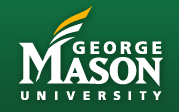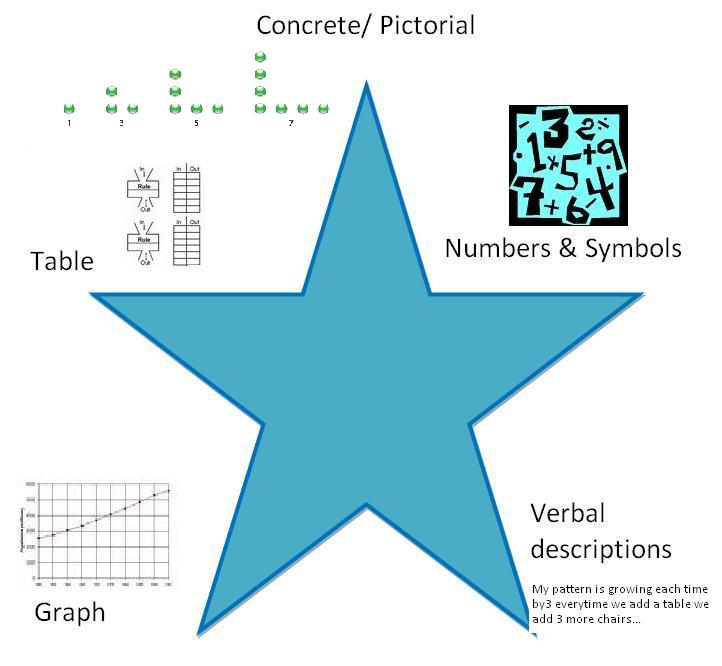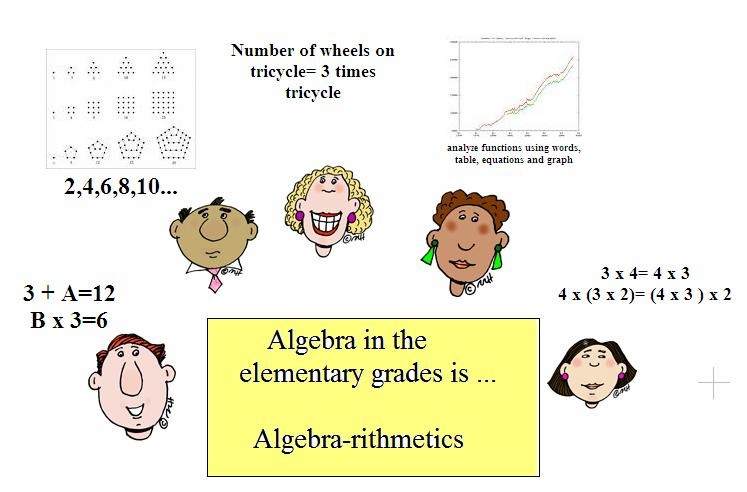### Improving  Mathematical Practices via Algebraic Connections & TechnologY

 About IMPACT IMPACT Connections Problems Powerpoints & Materials Instructional Practices Lesson Study Discussion Forum math tech- tools Resources & VideosBIG IDEAS for I.M.p.a.c.t. in math
1.  Rediscover the curriculum through Algebra Eyes and Ears
-Making generalizations
-Connecting big ideas within the curriculum
-Bringing out  curricular potential for algebraic connections

2. Develop Algebraic Habits of Mind
-abstracting from computations
-building rules from patterns
-doing and undoing

3. Engage diverse learners in meaningful math discourse

4. Leverage technology to build math knowledge

5. Establish a collaborative mentoring network through Lesson Study.Algebraic Concept Connections

• #### Grade 1 Curriculum Focal Points

Number and Operations and Algebra: Developing understandings of addition and subtraction and strategies for basic addition facts and related subtraction facts.
Children develop strategies for adding and subtracting whole numbers on the basis of their earlier work with small numbers. They use a variety of models, including discrete objects, length-based models (e.g., lengths of connecting cubes), and number lines, to model “part-whole,” “adding to,” “taking away from,” and “comparing” situations to develop an understanding of the meanings of addition and subtraction and strategies to solve such arithmetic problems. Children understand the connections between counting and the operations of addition and subtraction (e.g., adding two is the same as “counting on” two). They use properties of addition (commutativity and associativity) to add whole numbers, and they create and use increasingly sophisticated strategies based on these properties (e.g., “making tens”) to solve addition and subtraction problems involving basic facts. By comparing a variety of solution strategies, children relate addition and subtraction as inverse operations.

• Grade 2 Curriculum Focal Points

Number and Operations and Algebra: Developing quick recall of addition facts and related subtraction facts and fluency with multidigit addition and subtraction.

Children use their understanding of addition to develop quick recall of basic addition facts and related subtraction facts. They solve arithmetic problems by applying their understanding of models of addition and subtraction (such as combining or separating sets or using number lines), relationships and properties of number (such as place value), and properties of addition (commutativity and associativity). Children develop, discuss, and use efficient, accurate, and generalizable methods to add and subtract multidigit whole numbers. They select and apply appropriate methods to estimate sums and differences or calculate them mentally, depending on the context and numbers involved. They develop fluency with efficient procedures, including standard algorithms, for adding and subtracting whole numbers, understand why the procedures work (on the basis of place value and properties of operations), and use them to solve problems.
Algebra:
Children use number patterns to extend their knowledge of properties of numbers and operations. For example, when skip counting, they build foundations for understanding multiples and factors.

• #### Grade 3 Curriculum Focal Points

Number and Operations and Algebra: Developing understandings of multiplication anddivision and strategies for basic multiplication facts and related division facts.
Students understand the meanings of multiplication and division of whole numbers through the use of representations (e.g., equal-sized groups, arrays, area models, and equal “jumps” on number lines for multiplication, and successive subtraction, partitioning, and sharing for division). They use properties of addition and multiplication (e.g., commutativity, associativity, and the distributive property) to multiply whole numbers and apply increasingly sophisticated strategies based on these properties to solve multiplication and division problems involving basic facts. By comparing a variety of solution strategies, students relate multiplication and division as inverse operations. Algebra: Understanding properties of multiplication and the relationship between multiplication and division is a part of algebra readiness that develops at grade 3. The creation and analysis of patterns and relationships involving multiplication and division should occur at this grade level. Students build a foundation for later understanding of functional relationships by describing relationships in context with such statements as, “The number of legs is 4 times the number of chairs.”

• Grade 4 Curriculum Focal Points

Number and Operations and Algebra: Developing quick recall of multiplication facts and related division facts and fluency with whole number multiplication.
Students use understandings of multiplication to develop quick recall of the basic multiplication facts and related division facts. They apply their understanding of models for multiplication (i.e., equal sized groups, arrays, area models, equal intervals on the number line), place value, and properties of operations (in particular, the distributive property) as they develop, discuss, and use efficient, accurate, and generalizable methods to multiply multidigit whole numbers. They select appropriate methods and apply them accurately to estimate products or calculate them mentally, depending on the context and numbers involved. They develop fluency with efficient procedures, including the standard algorithm, for multiplying whole numbers, understand why the procedures work (on the basis of place value and properties of operations), and use them to solve problems.

Algebra: Students continue identifying, describing, and extending numeric patterns involving all operations and nonnumeric growing or repeating patterns. Through these experiences, they develop an understanding of the use of a rule to describe a sequence of numbers or objects.

•   Grade 5 Curriculum Focal Points

Number and Operations and Algebra: Developing an understanding of and fluency with division of whole numbers.
Students apply their understanding of models for division, place value, properties, and the relationship of division to multiplication as they develop, discuss, and use efficient, accurate, and generalizable procedures to find quotients involving multidigit dividends. They select appropriate methods and apply them accurately to estimate quotients or calculate them mentally, depending on the context and numbers involved. They develop fluency with efficient procedures, including the standard algorithm, for dividing whole numbers, understand why the procedures work (on the basis of place value and properties of operations), and use them to solve problems. They consider the context in which a problem is situated to select the most useful form of the quotient for the solution, and they interpret it appropriately.

Algebra: Students use patterns, models, and relationships as contexts for writing and solving simple equations and inequalities. They create graphs of simple equations. They explore prime and composite numbers and discover concepts related to the addition and subtraction of fractions as they use factors and multiples, including applications of common factors and common multiples. They develop an understanding of the order of operations and use it for all operations.

• Grade 6 Curriculum Focal Points

Algebra: Writing, interpreting, and using mathematical expressions and equations.
Students write mathematical expressions and equations that correspond to given situations, they evaluate expressions, and they use expressions and formulas to solve problems. They understand that variables represent numbers whose exact values are not yet specified, and they use variables appropriately. Students understand that expressions in different forms can be equivalent, and they can rewrite an expression to represent a quantity in a different way (e.g., to make it more compact or to feature different information). Students know that the solutions of an equation are the values of the variables that make the equation true. They solve simple one-step equations by using number sense, properties of operations, and the idea of maintaining equality on both sides of an equation. They construct and analyze tables (e.g., to show quantities that are in equivalent ratios), and they use equations to describe simple relationships (such as 3x = y) shown in a table.

Algebra: Students use the commutative, associative, and distributive properties to show that two expressions are equivalent. They also illustrate properties of operations by showing that two expressions are equivalent in a given context (e.g., determining the area in two different ways for a rectangle whose dimensions are x + 3 by 5). Sequences, including those that arise in the context of finding possible rules for patterns of figures or stacks of objects, provide opportunities for students to develop formulas.

• Grade 7 Curriculum Focal Points

Number and Operations and Algebra and Geometry: Developing an understanding of and applying proportionality, including similarity.
Students extend their work with ratios to develop an understanding of proportionality that they apply to solve single and multistep problems in numerous contexts. They use ratio and proportionality to solve a wide variety of percent problems, including problems involving discounts, interest, taxes, tips, and percent increase or decrease. They also solve problems about similar objects (including figures) by using scale factors that relate corresponding lengths of the objects or by using the fact that relationships of lengths within an object are preserved in similar objects. Students graph proportional relationships and identify the unit rate as the slope of the related line. They distinguish proportional relationships (y/x = k, or y = kx) from other relationships, including inverse proportionality (xy = k, or y = k/x).

Measurement and Geometry and Algebra: Developing an understanding of and using formulas to determine surface areas and volumes of three-dimensional shapes.
By decomposing two- and three-dimensional shapes into smaller, component shapes, students find surface areas and develop and justify formulas for the surface areas and volumes of prisms and cylinders. As students decompose prisms and cylinders by slicing them, they develop and understand formulas for their volumes (Volume = Area of base × Height). They apply these formulas in problem solving to determine volumes of prisms and cylinders. Students see that the formula for the area of a circle is plausible by decomposing a circle into a number of wedges and rearranging them into a shape that approximates a parallelogram. They select appropriate two- and three dimensional shapes to model real-world situations and solve a variety of problems (including multistep problems) involving surface areas, areas and circumferences of circles, and volumes of prisms and cylinders.

Number and Operations and Algebra: Developing an understanding of operations on all rational numbers and solving linear equations.
Students extend understandings of addition, subtraction, multiplication, and division, together with their properties, to all rational numbers, including negative integers. By applying properties of arithmetic and considering negative numbers in everyday contexts (e.g., situations of owing money or measuring elevations above and below sea level), students explain why the rules for adding, subtracting, multiplying, and dividing with negative numbers make sense. They use the arithmetic of rational numbers as they formulate and solve linear equations in one variable and use these equations to solve problems. Students make strategic choices of procedures to solve linear equations in one variable and implement them efficiently, understanding that when they use the properties of equality to express an equation in a new way, solutions that they obtain for the new equation also solve the original equation.

• Grade 8 Curriculum Focal Points

Algebra: Analyzing and representing linear functions and solving linear equations and systems of linear equations.
Students use linear functions, linear equations, and systems of linear equations to represent, analyze, and solve a variety of problems. They recognize a proportion (y/x = k, or y = kx) as a special case of a linear equation of the form y = mx + b, understanding that the constant of proportionality (k) is the slope and the resulting graph is a line through the origin. Students understand that the slope (m) of a line is a constant rate of change, so if the input, or x-coordinate, changes by a specific amount, a, the output, or y-coordinate, changes by the amount ma. Students translate among verbal, tabular, graphical, and algebraic representations of functions (recognizing that tabular and graphical representations are usually only partial representations), and they describe how such aspects of a function as slope and y-intercept appear in different representations. Students solve systems of two linear equations in two variables and relate the systems to pairs of lines that intersect, are parallel, or are the same line, in the plane. Students use linear equations, systems of linear equations, linear functions, and their understanding of the slope of a line to analyze situations and solve problems.

Geometry and Measurement: Analyzing two- and three-dimensional space and figures by using distance and angle.
Students use fundamental facts about distance and angles to describe and analyze figures and situations in two- and three-dimensional space and to solve problems, including those with multiple steps. They prove that particular configurations of lines give rise to similar triangles because of the congruent angles created when a transversal cuts parallel lines. Students apply this reasoning about similar triangles to solve a variety of problems, including those that ask them to find heights and distances. They use facts about the angles that are created when a transversal cuts parallel lines to explain why the sum of the measures of the angles in a triangle is 180 degrees, and they apply this fact about triangles to find unknown measures of angles. Students explain why the Pythagorean theorem is valid by using a variety of methods—for example, by decomposing a square in two different ways. They apply the Pythagorean theorem to find distances between points in the Cartesian coordinate plane to measure lengths and analyze polygons and polyhedra.

Data Analysis and Number and Operations and Algebra: Analyzing and summarizing data sets.
Students use descriptive statistics, including mean, median, and range, to summarize and compare data sets, and they organize and display data to pose and answer questions. They compare the information provided by the mean and the median and investigate the different effects that changes in data values have on these measures of center. They understand that a measure of center alone does not thoroughly describe a data set because very different data sets can share the same measure of center. Students select the mean or the median as the appropriate measure of center for a given purpose.

Algebra: Students encounter some nonlinear functions (such as the inverse proportions that they studied in grade 7 as well as basic quadratic and exponential functions) whose rates of change contrast with the constant rate of change of linear functions. They view arithmetic sequences, including those arising from patterns or problems, as linear functions whose inputs are counting numbers. They apply ideas about linear functions to solve problems involving rates such as motion at a constant speed.

http://www.math.ucla.edu/~kriegler/pub/algebrat.html